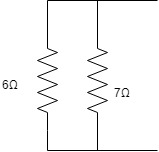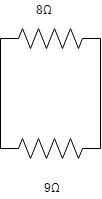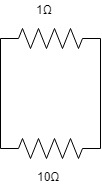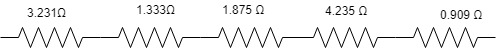# How to calculate Equivalent Resistance?

Equivalent Resistance of a circuit is obtained by calculating parallel and series elements in a circuit. A circuit may contain many series and parallel connected elements. In this topic on How to calculate Equivalent Resistance, we have to simplify the circuit elements.

To find the value of a resistor, a multimeter of Resistor Color Coding method can be used.

To calculate the Equivalent Resistance of the above circuit we need to simplify each part. In this example, start simplifying from the parallel resistor configuration.

The formula to use:

Rp = R1R2/ (R1 + R2)

Rs = R1 + R2

Let’s start to simplify from each part.Code Conversion | Excess-3 T0 Bcd Converter

In this part, the following resistors 6Ω are 7Ω connected in a parallel configuration.

Rp = 6 * 7/ (6 + 7)

Rp = 42/ 13

Rp = 3.2307

Rp = 3.231Ω

(Good practice is to round off three digits)Here the resistors 2Ω and 4Ω are connected in a parallel configuration.

Rp = 2 * 4/ (2 + 4)

Rp = 8 / 6

Rp = 1.3333

Rp = 1.333ΩMethods of Neutral Grounding | Neutral Earthing

Here the resistors 3Ω and 5Ω are connected in a parallel configuration.

Rp = 3 * 5 /(3 + 5)

Rp = 15 / 8

Rp = 1.875 ΩHere the 8Ω and 9Ω are connected in a parallel configuration.

Rp = 8 * 9 / (8 + 9)

Rp = 72/ 17

Rp = 4.235 ΩPower System Protection | Relay Roles

Here the following resistors 1Ω and 10Ω are connected in a parallel configuration.

Rp = 1 * 10/ (1 + 10)

Rp = 10/ 11

Rp = 0.909 Ω

Now add all the values which you got from simplifying the Parallel Configuration. The reason why we are adding is each and everything is connected in a series configuration.

Now the circuit is simplified as follows.Rs = 3.231Ω +  1.333Ω + 1.875 Ω +  4.235 Ω + 0.909 Ω

Rs = 11.583 Ω  which is also the.

Rab = 11.583 Ω

This example is applicable only for simple circuits. For circuits with more complexity, there were network reduction theorems. We can’t solve complex circuits only with this formula, This doesn’t mean we can’t solve but what I am saying is it was practically difficult to solve.

Here I have shown an example with 2 parallel resistors. For ‘n’ parallel resistors use this formula to reduce the network.

1/Rp = (1/R1) +(1/R2) + (1/R3)….. + (1/Rn)

or

Rp = R1R2R3Rn/ (R1 + R2 + R3 + Rn)

## You may also interested in: